# Bulk Specific Gravity

## Overview

The bulk specific gravity test is used to determine the specific gravity of a compacted HMA sample by determining the ratio of its weight to the weight of an equal volume of water.

The bulk specific gravity test measures a HMA sample’s weight under three different conditions (Figure 1):

• Dry (no water in sample).
• Saturated surface dry (SSD, water fills the HMA air voids).
• Submerged in water (underwater).

Using these three weights and their relationships, a sample’s apparent specific gravity, bulk specific gravity and bulk SSD specific gravity as well as absorption can be calculated.

HMA bulk specific gravity is needed to determine weight-volume relationships and to calculate various volume-related quantities such as air voids and voids in mineral aggregate (VMA).

The standard bulk specific gravity test is:

• AASHTO T 166: Bulk Specific Gravity of Compacted Bituminous Mixtures Using Saturated Surface-Dry Specimens
• ASTM D 2726: Bulk Specific Gravity and Density of Non-Absorptive Compacted Bituminous Mixtures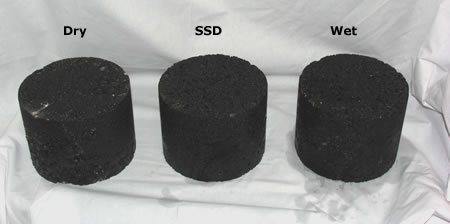Figure 1. HMA samples in three conditions.

## Background

Specific gravity is a measure of a material’s density (mass per unit volume) as compared to the density of water at 73.4°F (23°C). Therefore, by definition, water at 73.4°F (23°C) has a specific gravity of 1.

### Bulk Specific Gravity Use

Superpave mix design is a volumetric process; key properties are expressed in terms of volume. However, direct volume measurements are difficult, therefore weight measurements are usually made and then converted to a volume based on material specific gravities. Bulk specific gravity is involved in most key mix design calculations including air voids, VMA and, indirectly, VFA. Correct and accurate bulk specific gravity determinations are vital to proper mix design. An incorrect bulk specific gravity value will result in incorrectly calculated air voids, VMA, VFA and ultimately result in an incorrect mix design.

### Methods of Determining Bulk Specific Gravity

Although the Test Description section describes the standard AASHTO T 166 saturated surface dry (SSD) water displacement method, there are a number of other methods available. Each one uses a slightly different way to determine specimen volume and may result in different bulk specific gravity values.

#### Water Displacement Methods

These methods, based on Archimedes Principle, calculate specimen volume by weighing the specimen (1) in a water bath and (2) out of the water bath. The difference in weights can then be used to calculate the weight of water displaced, which can be converted to a volume using the specific gravity of water.

##### Saturated Surface Dry (SSD)

The most common method (and the one described in the Test Description section), calculates the specimen volume by subtracting the mass of the specimen in water (Figure 2) from the mass of a SSD specimen. SSD is defined as the specimen condition when the internal air voids are filled with water and the surface (including air voids connected to the surface) is dry. This SSD condition allows for internal air voids to be counted as part of the specimen volume and is achieved by soaking the specimen in a water bath for 4 minutes then removing it and quickly blotting it dry with a damp towel.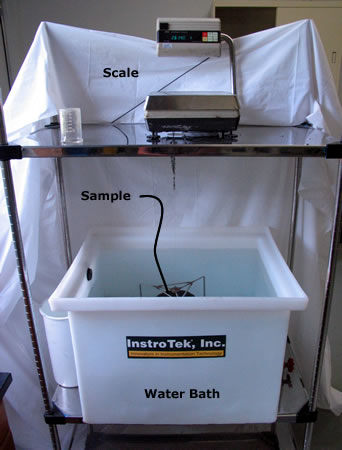Figure 2. SSD Method.

[warning]One critical problem with this method is that if a specimen’s air voids are high, and thus potentially interconnected (for dense-graded HMA this occurs at about 8 to 10 percent air voids), water quickly drains out of them as the specimen is removed from its water bath, which results in an erroneously low HMA sample volume measurement and thus an erroneously high bulk specific gravity.[/warning]

##### Paraffin

This method determines volume similarly to the water displacement method but uses a melted paraffin wax instead of water to fill a specimen’s internal air voids (Figure 3). Therefore, after the wax sets there is no possibility of it draining out and, theoretically, a more accurate volume can be calculated. In practice, the paraffin is difficult to correctly apply and test results are somewhat inconsistent.Figure 3. Parafin-covered HMA sample.
##### Parafilm

In this method the specimen is wrapped in a thin paraffin film (Figure 4) and then weighed in and out of water. Since the specimen is completely wrapped when it is submerged, no water can get into it and a more accurate volume measurement is theoretically possible. However, in practice the paraffin film application is quite difficult and test results are inconsistent.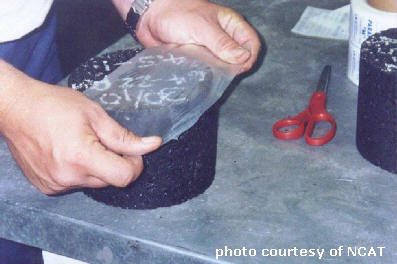Figure 4: Covering a HMA sample with Parafilm.
##### CoreLok

This method calculates specimen volume like the parafilm method but uses a vacuum chamber (Figure 5) to shrink-wrap the specimen in a high-quality plastic bag (Figure 6) rather than cover it in a paraffin film (Video 1). This method has shown promise in both accuracy and precision.Figure 5: CoreLok vacuum chamber with sample inside.Figure 6: CoreLok sample vacuum sealed in a plastic bag.
http://youtu.be/MtrPgvC8XDwVideo 1: CoreLok device.

#### Dimensional

This method, the simplest, calculates the volume based on height and diameter/width measurements. Although it avoids problems associated with the SSD condition, it is often inaccurate because it assumes a perfectly smooth surface, thereby ignoring surface irregularities (i.e., the rough surface texture of a typical specimen).

#### Gamma Ray

The gamma ray method is based on the scattering and absorption properties of gamma rays with matter. When a gamma ray source of primary energy in the Compton range is placed near a material, and an energy selective gamma ray detector is used for gamma ray counting, the scattered and unscattered gamma rays with energies in the Compton range can be counted exclusively. With proper calibration, the gamma ray count is directly converted to the density or bulk specific gravity of the material (Troxler, 2001). Figure 7 shows the Troxler device.Figure 7: Troxler Model 3660 CoreReader.

## Test Description

The following description is a brief summary of the test. It is not a complete procedure and should not be used to perform the test. The complete procedure can be found in:

• AASHTO T 166: Bulk Specific Gravity of Compacted Asphalt Mixtures Using Saturated Surface-Dry Specimens
• ASTM D 2726: Bulk Specific Gravity and Density of Non-Absorptive Compacted Bituminous Mixtures

Other standard tests available to determine bulk specific gravity that are not described in this section are:

• AASHTO T 275: Bulk Specific Gravity of Compacted Bituminous Mixtures Using Paraffin-Coated Specimens
• AASHTO TP 69: Bulk Specific Gravity and Density of Compacted Asphalt Mixtures Using Automatic Vacuum Sealing Method

### Summary

A compacted HMA sample (usually a SGC compacted laboratory sample or a field-obtained HMA core) is weighed dry, saturated surface dry (SSD) and submerged (Figure 1). These weights are used to calculate specific gravity and the percentage of water absorbed by the sample.

### Approximate Test Time

Each test takes approximately 7 minutes to conduct excluding preparation time. When several samples are tested the test time per sample can be reduced. Considerable preparation time may be necessary if contamination must be removed from the bottom of the sample.

### Basic Procedure

1. Dry specimen to a constant mass and cool to room temperature.

[note]Laboratory samples are typically dry at the beginning of the test; however, field samples will typically be damp.[/note]

1. Record the dry mass (Figure 8).Figure 8: Sample weighing.
1. Submerge sample in 77°F (25°C) water for 4 minutes and record the submerged mass . This can be done with a water-filled container on top of a scale or with a basket suspended in water under a scale (Figure 2).
2. Quickly blot the sample with a damp towel and record the surface dry mass.
[warning]Any water that escapes from the sample during weighing is considered part of the saturated specimen. If this water is not weighed, significant error can result.[/warning]

## Results

### Parameters Measured

Bulk specific gravity (Gmb) and the percentage of water absorbed by volume.

### Specifications

There is no specification for bulk specific gravity, but it is used to calculate other specified parameters such as air voids, VMA and VFA.

### Typical Values

Typical values for bulk specific gravity range from 2.200 to 2.500 depending upon the bulk specific gravity of the aggregate, the asphalt binder content, and the amount of compaction.

Absorption should typically be below 2 percent. If more than 2 percent water by volume is absorbed by the sample then this method is not appropriate. In this case, use AASHTO T 275, Bulk Specific Gravity of Compacted Bituminous Mixtures Using Paraffin-Coated Specimens or AASHTO TP 69, Bulk Specific Gravity and Density of Compacted Asphalt Mixtures Using Automatic Vacuum Sealing Method.

### Calculations (Interactive Equation)

Three different masses are recorded during the test. Their common symbols are:

A = mass of sample in air (g)
B = mass of SSD sample in air (g)
C = mass of sample in water (g)

These masses are used to calculate bulk specific gravity and water absorption using the following equations: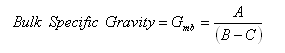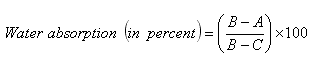[warning]Certainly, the accuracy of all measurements is important. However, of specific concern is the mass of the SSD sample. As mentioned in the background section, if a specimen’s air voids are high, and thus potentially interconnected (for dense-graded HMA this occurs at about 8 to 10 percent air voids), water quickly drains out of them as the specimen is removed from its water bath, which results in an erroneously low SSD weight, which leads to an erroneously low HMA sample volume measurement and thus an erroneously high bulk specific gravity.[/warning]

Footnotes    (↵ returns to text)
1. Troxler Electronic Laboratories, Inc. (Troxler).  (March 2001).  Measuring Bulk Specific Gravity of Compacted Specimens Using The Troxler Model 3660 CoreReader.  Web page on the Troxler web site.  Troxler Electronic Laboratories, Inc.  Research Triangle Park, NC. Accessed 1 July 2002.